# Cone (geometry)

A cone is a three-dimensional geometric shape consisting of all line segments joining a single point (the apex or vertex) to every point of a two-dimensional figure (the base). The term cone sometimes refers to just the lateral surface of a solid cone, that is, the locus of all line segments that join the apex to the perimeter of the base.

The line joining the apex of the cone to the center of the base (suitably defined) is called the axis. In common usage and in elementary geometry, the base is a circle, and the axis is perpendicular to the plane of the base. Such a cone is called a right circular cone.

## Contents

When a right circular conical surface is intersected by a plane, the curve produced at the intersection is called a conic section (or conic). Circles, ellipses, parabolas, and hyperbolas are all conic sections. The study of cones and conic sections is important not only for mathematics and physics but also for a variety of engineering applications.

## Elements and special cases

The perimeter of the base is called the directrix, and each of the line segments between the directrix and apex is a generatrix of the lateral surface.

In general, the base of a cone may have any shape, and the apex may lie anywhere. However, it is often assumed that the base is bounded and has nonzero area, and that the apex lies outside the plane of the base.

Circular cones and elliptical cones have circular and elliptical bases, respectively. A pyramid is a special type of cone with a polygonal base.

If the axis of the cone is at right angles to the base then it is said to be a "right cone"; otherwise, it is an "oblique cone."

A cone with its apex cut off by a plane parallel to its base is called a truncated cone or frustum.

The base radius of a circular cone is the radius of its base; often this is simply called the radius of the cone.

The aperture of a right circular cone is the maximum angle between two generatrix lines; if the generatrix makes and angle θ to the axis, the aperture is 2θ.

### Infinite and doubly infinite cones

In mathematical usage, the word cone is also used for an infinite cone, which is the union of any set of half-lines that start at a common apex point. This type of cone does not have a bounding base and extends to infinity. A doubly infinite cone (or double cone) is the union of any set of straight lines that pass through a common apex point, and therefore extends symmetrically on both sides of the apex. Depending on the context, the word may also mean specifically a convex cone or a projective cone. The boundary of an infinite or doubly infinite cone is a conical surface. For infinite cones, the word axis usually refers to the axis of rotational symmetry (if any).

## Formulas

The volume$V$ of any conic solid is one third the area of the base$b$ times the height$h$ (the perpendicular distance from the base to the apex).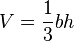$V = \frac{1}{3} b h$

The center of mass of a conic solid is at 1/4 of the height on the axis.

### Right circular cone

For a circular cone with radius r and height h, the formula for volume becomes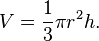$V = \frac{1}{3} \pi r^2 h.$

For a right circular cone, the surface area$A$ is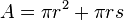$A =\pi r^2 + \pi r s\,$   where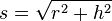$s = \sqrt{r^2 + h^2}$   is the slant height.

The first term in the area formula,$\pi r^2$, is the area of the base, while the second term,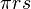$\pi r s$, is the area of the lateral surface.

A right circular cone with height$h$ and aperture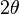$2\theta$, whose axis is the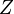$Z$ coordinate axis and whose apex is the origin, is described parametrically as$S(s,t,u) = (u \tan s \cos t, u \tan s \sin t, u)$

where$s,t,u$ range over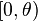$[0,\theta)$,$[0,2\pi)$, and$[0,h]$, respectively.

In implicit form, the same solid is defined by the inequalities$\{ S(x,y,z) \leq 0, z\geq 0, z\leq h\}$,

where$S(x,y,z) = (x^2 + y^2)(\cos\theta)^2 - z^2 (\sin \theta)^2\,$.

More generally, a right circular cone with vertex at the origin, axis parallel to the vector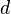$d$, and aperture$2\theta$, is given by the implicit vector equation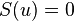$S(u) = 0$ where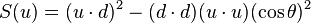$S(u) = (u \cdot d)^2 - (d \cdot d) (u \cdot u) (\cos \theta)^2$   or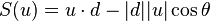$S(u) = u \cdot d - |d| |u| \cos \theta$

where$u=(x,y,z)$, and$u \cdot d$ denotes the dot product.